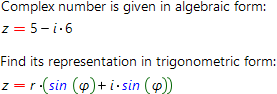# Complex number form converterThis calculator allows one to convert complex number from one representation form to another with step by step solution. For example, you can convert complex number from algebraic to trigonometric representation form or from exponential back to algebraic, ect. To use the calculator, one need to choose representation form of complex number and input data to the calculator. Not only numbers and fractions are allowed as inputs, but also the symbols (parameters) are accepted. Below is given some theoretical background of the different representation forms of complex numbers.

Expression

z=x+iy,

where x, y - arbitrary real numbers is called algebraic form of complex number.

Expression

z=r(cosφ + isinφ) (r0),

whereis called trigonometric form of complex number.

Finally, using the Euler formula:

e i φ = cosφ + isinφ ,

one can get exponential representation of complex number:

z=r e iφ

Convert complex number from one representation to another